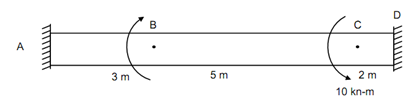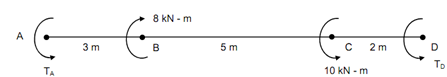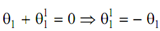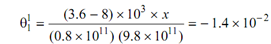## Angle of twist is zero on the shaft, Mechanical Engineering

Assignment Help:

Angle of twist is zero on the shaft:

A shaft 10 m long and 100 mm diameter during its length is fixed at the ends and is subjected to two opposite torques of 8 kN-m and 10 kN-m at a distance of 3 m and 8 m from one end. Discover the fixing torques at the ends. What is the maximum angle of twist and the maximum shear stress in the shaft? At what section the angle of twist is zero on the shaft?

Take E = 80 GPa.

Solution

Σ T = 0

⇒  TA  - 8 + 10 + TD  = 0

∴          TA  + TD  = - 2

G = 80 GPa = 0.8 × 1011 N/m2              -------------- (1)

d = 100 mm = 0.1 m

J =  (π /32)d 4 = (π/32) × (0.1)4  = 9.8 × 10- 6 m4

R = d /2= 100/2 = 0.05 m ,   ZP = 1.96 × 10- 4 m3Figure (a)Figure (b) : Line Diagram

Portion AB

θ 1 = (TA . l )/GJ = (TA × 3 )/GJ                         ---------- (2)

Portion BC

θ2 = ((TA  - 8) × 5 )/     GJ               -------- (3)

Portion CD

θ3 = ((TA - 8 + 10) × 2)/ GJ = ((TA + 2) × 2)/ GJ                      ---------- (4)

Angle of twist at D co.r.t A = 0

(TA × 3 )/ G J + ((TA  - 8) × 5 )/ GJ + ((TA  + 2) × 2)/ GJ = 0

∴          TA  = 3.6 kN-m                                       ----------- (5)

From Eqs. (1) and (5),

3.6 + TD = - 2

TD = - 5.6 kN-m = 5.6 kN-m    (  )

Angle of Twist

θ1 = ((3.6 × 103 ) × 3) / ((0.8 × 1011 ) (9.8 × 10- 6 ))

= 1.4 × 10- 2 radians

θ2 =      ((3.6 - 8) × 103 × 5 )/(0.8 × 1011 ) (9.8 × 10- 6 )= - 2.8 × 10- 2 radians

θ3 =      (3.6 + 2) × 103  × 2 /((0.8 × 1011 ) (9.8 × 10- 6 ))= 1.4 × 10- 2 radians

θAB  = θ1 = 1.4 × 10- 2 radians

θ AC  = θ1 + θ2  = 1.4 × 10- 2 - 2.8 × 10- 2 = - 1.4 × 10- 2 radians

θAD  = θAC  + θ3 = - 1.4 × 10- 2

Shear Stress

+ 1.4 × 10- 2  = 0

τ max     = (T/ J) . R =  T/ Z P

Portion AB

τmax = 3.6 × 103 / (1.96 × 10- 4) = 18.4 × 106 N/m2  = 18.4 N/mm2

Portion BC

τ max = (3.6 - 8) × 103/ (1.96 × 10- 4)

= - 22.4 × 106 N/m2  = - 22.4 N/mm2

Portion CD

τ max      = (3.6 + 2) × 103/1.96 × 10- 6 = 28.6 × 106 N/m2  = 28.6 N/mm2

Zero Angle of Twist

It occurs between B and C

θ1 = 1.4 × 10- 2 radians∴ x = 2.5 m from B.

#### Evaluate elongation of wire, Evaluate Elongation of wire: An aerial c...

Evaluate Elongation of wire: An aerial copper wire ( E =1 x 10 5 N/m m 2 ) 40 m long is having cross sectional area of 80 mm 2 and weighs 0.6 N per meter run. I

#### Plate clutch movement on center clutch-clutch jerking, Plate clutch movemen...

Plate clutch movement on center clutch : Place plate clutch on centre clutch as shown in the picture and move the plate clutch up and down, while moving the plate clutch in the ce

#### Explain concept of a corner or angled window, Question 1: Retail design...

Question 1: Retail design tends to be rapid turnover business. Your potential client needs a window display for his retail sports wear shop for the summer sales. The assumed bu

#### Shielded metal arc welding (smaw), SHIELDED METAL ARC WELDING (SMAW) It...

SHIELDED METAL ARC WELDING (SMAW) It also known as Manual Metal Arc Welding (MMAW). The heat required for welding is generated by the electric arc formed between metallic elect

#### Powder metallurgy, primary steps in PM

primary steps in PM

#### Relation between stress and strain, Relation between stress and strain: ...

Relation between stress and strain: The relation between stress and strain is generally shown by plotting a stress-strain (σ -e) diagram. Stress can be plotted on ordinate (ve

#### Strength of materials, sir/madam, i would like to teach online how can i ha...

sir/madam, i would like to teach online how can i have to proceed

#### Maximum bending moment, Maximum Bending Moment: The SF changes sign at...

Maximum Bending Moment: The SF changes sign at A, among A and B and at B. Let a section XX at a distance x from the end C as illustrated in Figure SF at section XX, F x

#### Analysis of trusses, State the assumptions in pin joined trusses?

State the assumptions in pin joined trusses?

#### Compound BArs, three vertical, parallel straight rods, each 2.6m in length ...

three vertical, parallel straight rods, each 2.6m in length have their upper ends firmly secured and their lower supporting a rigid horizontal bar with a weight of 8KN, which cause